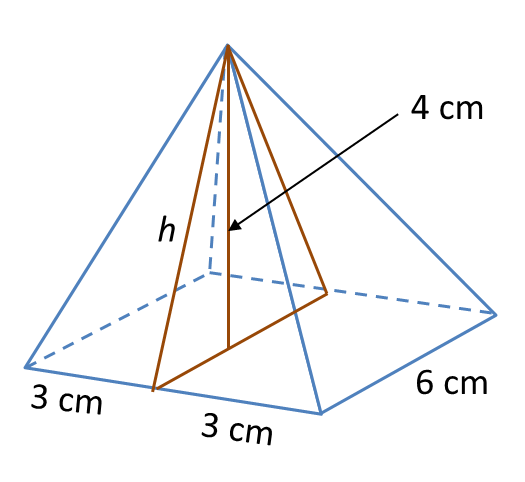# 12.2.2 Solid Geometry (II), PT3 Focus Practice

                         
12.2.2 Solid Geometry (II), PT3 Focus Practice
 
  Question 5:
  Sphere below has a surface area of 221.76 cm2.Calculate its radius.
                          $\left(\pi =\frac{22}{7}\right)$                        
 
  Solution:
  Surface area of sphere = 4πr2
                          $\begin{array}{l}4\pi {r}^{2}=221.76\\ 4×\frac{22}{7}×{r}^{2}=221.76\\ {r}^{2}=\frac{221.76×7}{4×22}\\ {r}^{2}=17.64\\ r=\sqrt{17.64}\\ r=4.2\text{cm}\end{array}$                      
 
 
 
                         
Question 6:
  Diagram below shows a right pyramid with a square base.Given the height of the pyramid is 4 cm.
  Calculate the total surface area, in cm2, of the right pyramid.
 
  Solution:h2 = 32 + 42
  = 9 + 16
  = 25
  h = √25   = 5 cm2
 
  Total surface area of the right pyramid
  = Base area + 4 (Area of triangle)
  = (6 × 6) + 4 × 4 (½ × 6 × 5)
  = 36 + 60
  = 96 cm2
 
 
 
         
Question 7:
 
Diagram below shows a prism.Draw to full scale, the net of the prism on the grid in the answer space. The grid has equal squares with sides of 1 unit.
 
 Solution:# 12.2.1 Solid Geometry (II), PT3 Focus Practice

12.2.1 Solid Geometry (II), PT3 Focus Practice

Question 1:
Diagram below shows closed right cylinder.

Calculate the total surface area, in cm2, of the cylinder.

$\left(\pi =\frac{22}{7}\right)$

Solution
:
Total surface area
= 2(πr2) + 2πrh
$\begin{array}{l}=\left(2×\frac{22}{7}×{7}^{2}\right)+\left(2×\frac{22}{7}×7×20\right)\\ =308+880\\ =1188c{m}^{2}\end{array}$

Question 2:
Diagram below shows a right prism with right-angled triangle ABC as its uniform cross section.
Calculate the total surface area, in cm2, of the prism.

Solution
:
$\begin{array}{l}AB=\sqrt{{5}^{2}-{3}^{2}}\\ =\sqrt{25-9}\\ =\sqrt{16}\\ =4cm\end{array}$

Total surface area
= 2 (½× 3 × 4) + (3 × 10) + (4 × 10) + (5 × 10)
= 12 + 30 + 40 + 50
= 132 cm2

Question 3:
Diagram below shows a right pyramid with a square base.

Calculate the total surface area, in cm2, of the right pyramid.

Solution
:
h2= 102 – 62
= 100 – 36
= 64
= √64
= 8cm

Total surface area of the right pyramid
= (12 × 12) + 4 × (½× 12 × 8)
= 144 + 192
= 336 cm2

Question 4:
The diagram shows a cone. The radius of its base is 3.5 cm and its slant height is 6 cm. Find the area of its curved surface.
( π= 22 7 )

Solution
:
Area of curved surface
= π × radius of base × slant height
= πrs
$\begin{array}{l}=\frac{22}{7}×3.5×6\\ =66c{m}^{2}\end{array}$